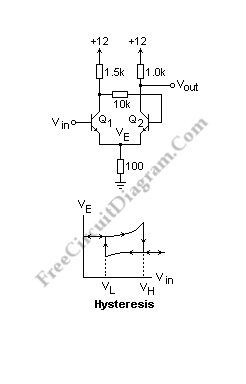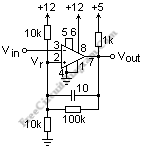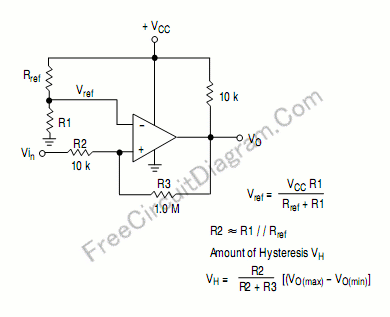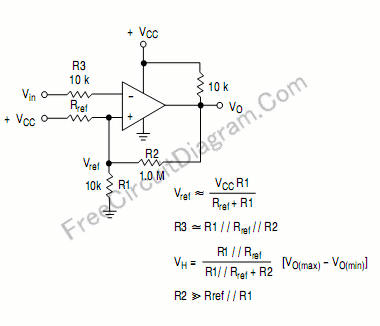## Discrete (Transistors) Schmitt TriggerAnother circuit (from Horowitz and Hill) is shown below. The circuit is almost the same except that the base of Q2 is not connected to the ground. The voltage at the emitters, Ve, is the important thing here. Q1 is off and the output goes low when the base of Q1 rises to 0.7V above Ve if the input is […]

## LM311 Comparator with HysteresisAn LM311 comparator circuit with the pin numbers  is shown in the schematic diagram figure below. The ground for this transistor is at pin 1 and the open collector output is at pin 7. It is shown pulled up to the logic voltage of +5 with a 1k resistor, which is typical. The LM311 output can sink 8 mA. A […]

## Non-Inverting Comparator With HisteresisThis circuit has similar function with inverting comparator with hysteresis, but the  output is not inverted. The output will be high if the input is higher than the upper threshold point, and the output will be low if the input is below the lower threshold point. Here is the circuit’s schematic diagram: What will happen if the input is between […]